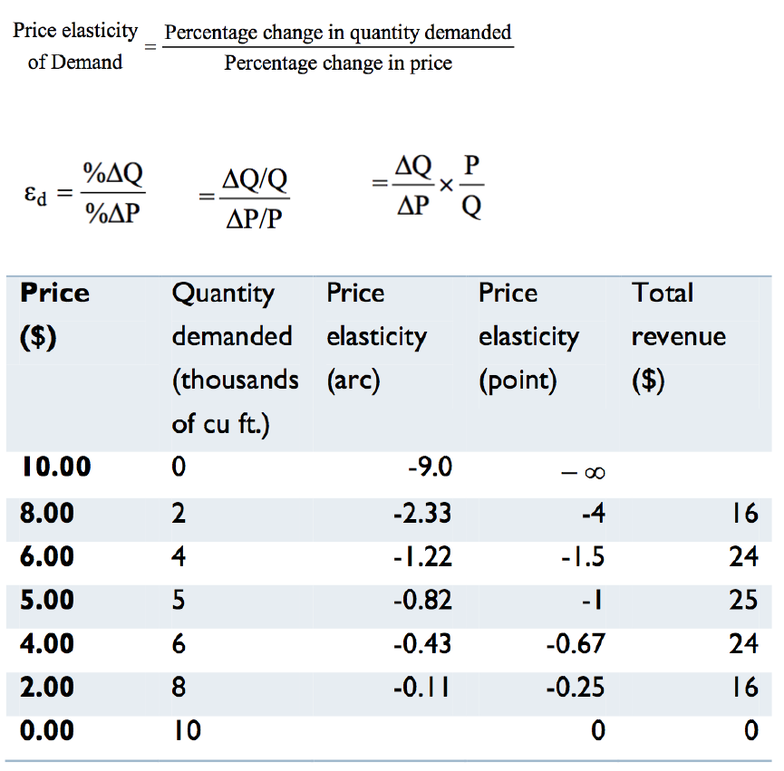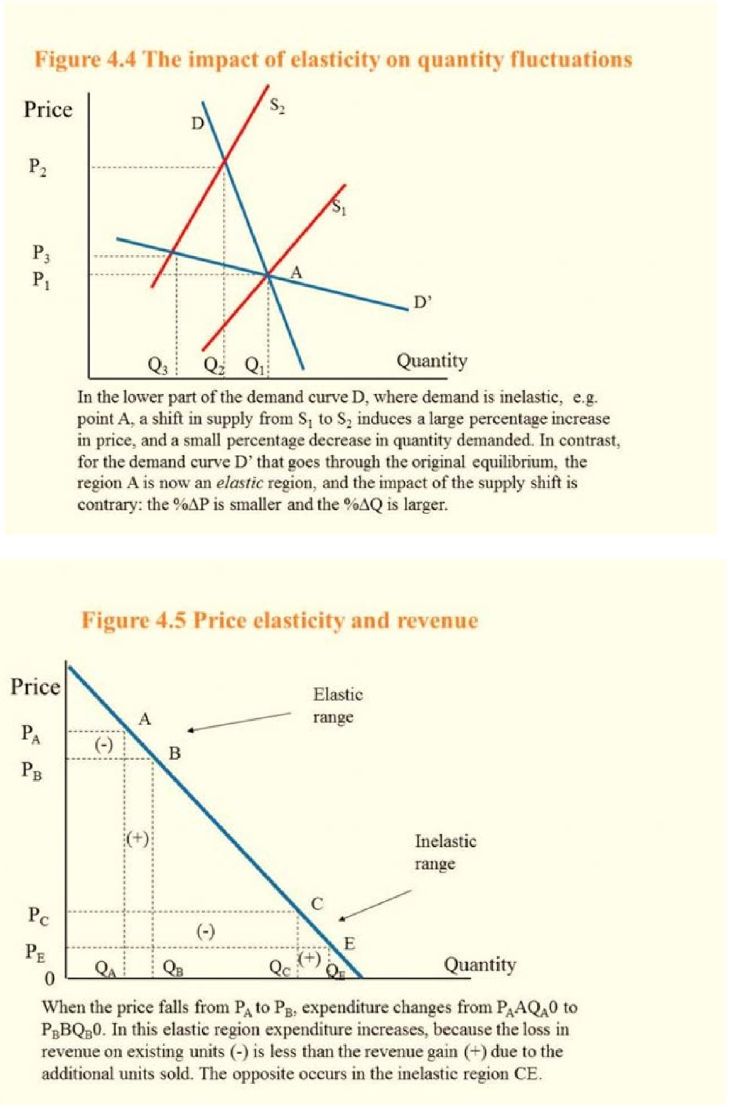Textbook Notes (270,000)
CA (160,000)
Concordia (1,000)
ECON (40)
Chapter 4

# ECON 201 Chapter 4: chapter 4 + questions.docx

Department
Economics
Course Code
ECON 201
Professor
Nahid Masoudi
Chapter
4

This preview shows pages 1-2. to view the full 6 pages of the document.The price elasticity of demand
is measured as the percentage change in quantity demanded,
divided by the percentage change in price
-9.0 = (0-2)/(10-8) * (10+8)/(0-2) – arc elasticity
-1.5 = (2-4)/(8-6) * 6/4 – point elasticity
-1 = (4-5)/(6-5) * 5/5 – point elasticity
-0.43 = (5-6)/(5-4) * (5+4)/(5+6) – arc elasticity
The arc elasticity of demand defines consumer responsiveness over a
segment or arc of the demand curve
The point elasticity of demand is the elasticity computed at a
particular point on the demand curve

Only pages 1-2 are available for preview. Some parts have been intentionally blurred.SLOOOOOOPE
Demand is elastic if the price elasticity is greater than unity. It is
inelastic if the value lies between unity and 0. It is unit elastic if the
value is exactly one